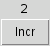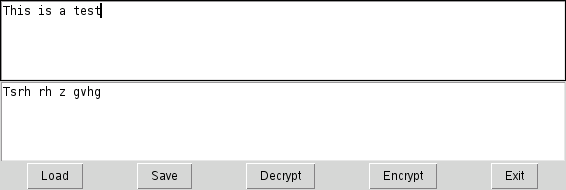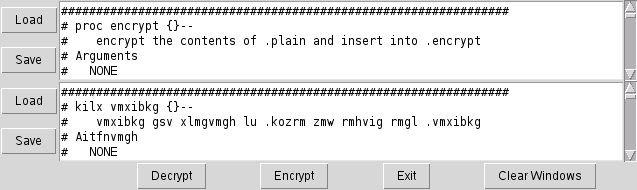## Lab 4: Encryption with lists and procedures.

1. Write a program that has one button and one label.
• The label should display the contents of a variable. (use `-textvariable`)
• The variable contains a number (initialized to 0).
• When you click the button, it invokes a procedure that adds 1 to the variable. Don't forget the scoping rules for local/global scope.
The results should resemble this (after clicking the Incr button a few times.2. The fibonacci series is generated by

1. Initialize a list as {1 1}
2. Create each new element by adding the end and end-1 elements of the list
ie: {1 1 (1+1=2)} then {1 1 2 (1+2=3)}, etc.
3. This can be implemented with three variables. Two to hold the previous two elements of the list, and one to hold the new sum.

Modify the previous program to display the next fibonacci number in a series each time you click the button.

All you need to change is the contents (and perhaps name) of the procedure.

3. One more modification to the previous program. Rewrite the procedure so that it adds a random integer between 0 and 9 to the previous value.

Run it a couple of times. Do you get the same set of numbers each time?

Most computer applications use a pseudo-random number generator that uses some fancy math to calculate a random number.

The number isn't actually random, it's just a random distribution. If you can force the random number generator to start at a known value, you'll always get the same values after that.

This is useful when you are debugging an application that uses random numbers and you want it to behave consistently.

The `srand()` function sets the random number generator start point. The Tcl interpreter calls this with the current time-in-seconds when it starts. This ensures that you always get a different pattern of random numbers when you run an application.

A line like this will force the random number generator to start from 0.

``````
expr srand(0)
``````

Add this to the program and check that you get the same random numbers each time you run it.

4. Here is the complete code from the lecture example.

The variable `alpha` is defined within the `encrypt` procedure. That makes it local to the `encrypt` procedure.

``````
################################################################
# proc encrypt {}--
#    encrypt the contents of .plain and insert into .encrypt
# Arguments
#   NONE
#
# Results
#   .encrypt window is modified.
#
proc encrypt {txt} {
# Fill the lookup table
set alpha \
{a b c d e f g h i j k l m n o p q r s t u v w x y z}

# Iterate through characters
foreach char [split \$txt {}] {
set pos [lsearch \$alpha \$char]
# If character is in list, encrypt it, else keep the character
if {\$pos >= 0} {
set pos2 [expr {(\$pos + 13) %26}]
set char2 [lindex \$alpha \$pos2]
} else {
set char2 \$char
}
append encrypt \$char2
}
return \$encrypt
}

################################################################
# proc encryptWin {inWin outWin}--
#    Read characters from inWin and insert encrypted characters
# into outWin
# Arguments
#   inWin:	Name of a text widget to read input from
#   outWin:	Name of a text widget to write encrypted text to
# Results
#   outWin widget contents are modified.
#
proc encryptWin {inWin outWin} {
set txt [\$inWin get 0.0 end]
\$outWin delete 0.0 end
\$outWin insert 0.0 [encrypt \$txt]
}

set w [text .plain]
grid \$w -columnspan 3
set w [text .encrypt]
grid \$w -columnspan 3

set w1 [button .b_encrypt -text "Encrypt" -command encryptWin .plain .encrypt ]
set w2 [button .b_exit -text "Exit" -command exit]
grid \$w1 \$w2

``````

This version of the `encrypt` procedure defines the `alpha` variable in the global scope. That way it only needs to be defined once, which makes the `encrypt` procedure more efficient. (The assignment is done once, instead of every time the `encrypt` procedure is invoked.

Replace the previous encrypt procedure with this one and test it.

This code won't work - fix it.

``````
# Fill the lookup table
set alpha {a b c d e f g h i j k l m n o p q r s t u v w x y z}

################################################################
# proc encrypt {}--
#    encrypt the contents of .plain and insert into .encrypt
# Arguments
#   NONE
#
# Results
#   .encrypt window is modified.
#
proc encrypt {txt} {

# Iterate through characters
foreach char [split \$txt {}] {
set pos [lsearch \$alpha \$char]
# If character is in list, encrypt it, else keep the character
if {\$pos >= 0} {
set pos2 [expr {(\$pos + 13) %26}]
set char2 [lindex \$alpha \$pos2]
} else {
set char2 \$char
}
append encrypt \$char2
}
return \$encrypt
}
``````

5. The previous `encrypt` procedure uses a fixed offset (Caesar) cipher.

Modern ciphers like DES use a random number and a secret seed to change the offset for each character.

Rework the `encrypt` procedure to use `expr srand(\$seed)` and `rand()` to generate a better encryption.

Write a decrypt procedure to convert back to plaintext to test this.

6. The previous encryption procedures used a single list and calculated offsets. We can write an encryption procedure that uses two lists - one for the plain text, and one for the cipher text:

``````

set plainChars   {a b c d e f g h i j k l m n o p q r s t u v w x y z}
set encryptChars {z y x w v u t s r q p o n m l k j i h g f e d c b a}

proc encrypt {txt} {
global plainChars
global encryptChars

foreach char [split \$txt {}] {
set pos [lsearch \$plainChars \$char]
# If character is in list, encrypt it, else keep the character
if {\$pos >= 0} {
set char2 [lindex \$encryptChars \$pos}
} else {
set char2 \$char
}
append encrypt \$char2
}
return \$encrypt
}
``````

This code has a small typo bug in it.

Cut/Paste it into your program and fix the bug, then write a decrypt procedure to convert cipherText into plainText.

7. The previous example uses a fixed relationship between plainText and cipherText. It always converts an 'a' to a 'z', etc.

The `encryptChars` array can be created on the fly with code like this

``````
################################################################
# proc buildEncryptChars {orig}--
#    return a list of reordered characters
# Arguments
#   orig	A list of list elements to be reordered.
#
# Results
#   No side effects.
#
proc randomizeList {orig} {
set origLen [llength \$orig]
for {set i 0} {\$i < \$origLen} {incr i} {
set pos [expr {int(rand()*[llength \$orig])}]
lappend new [lindex \$orig \$pos]
set orig [lreplace \$orig \$pos \$pos]
}
return \$new
}
``````

Modify the previous example by adding this procedure and then adding a wrapper around the `randomizeList` procedure to initialize the `encryptChars` variable.

The new procedure should accept a seed value so that you can decrypt a message later.

8. A useful encrypting/decryption program would allow you to load text from a file and then encrypt or decrypt it.

Add a Load button to this procedure that will call a procedure that reads a set of text and inserts it into the upper text widget so that you can then encrypt or decrypt it.

Add a Save button that will use the `tk_getSaveFile` command to get a filename, open the file in write mode, and save the data from the lower text window.

The results should look like this:9. An even more useful application would have buttons to Load and Save from each text window, a button to clear the text and scrollbars attached to the windows.

Add an argument to the Load and Save procedures in the previous example to select the window that will have data loaded to or saved from.

Modify the `buildGUI` procedure to create separate Load and Save buttons for each text window to load/save into that window.

Also modify the buildGUI procedure to contain scrollbars attached to each text widget.

The results should look something like this:10. Instead of splitting the incoming text into characters, it can be kept as words.

In that case, we can use two lists of words to generate a code message instead of a cipher message.

``````
set plain {IBM       Microsoft Buy  Sell Stock}
set code {{Blue Dog} Gate      Bake Bury Cake}
``````

Write the procedures so that the phrase

``````
If IBM is higher than Microsoft, buy their Stock
``````
becomes
``````
If Blue Dog is higher than Gate, Bake their Cake
``````

No online solution for this one. See if you can figure it out.